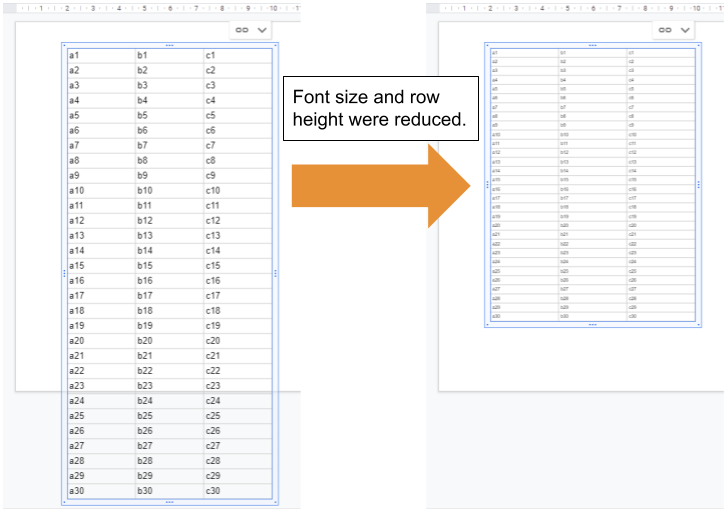Gists

This is a sample script for reducing the table height of the table inserted from Google Spreadsheet to Google Slides using Google Apps Script.## Sample script

Please copy and paste the following script to the script editor of Google Slides. This sample script uses Slides API. So, please enable Slides API at Advanced Google services. Ref

As the sample situation, this script supposes that a table is manually copied from Google Spreadsheet to the 1st slide of Google Slides. So when you test this script, please copy the cells from Google Spreadsheet to the 1st slide of Google Slides and run the function.

function myFunction() {
const fontSize = 5; // pt
const rowHeight = 10; // pt

const s = SlidesApp.getActivePresentation();
const slide = s.getSlides();
const table = slide.getTables();
for (let r = 0; r < table.getNumRows(); r++) {
const row = table.getRow(r);
for (let c = 0; c < row.getNumCells(); c++) {
row.getCell(c).getText().getTextStyle().setFontSize(fontSize);
}
}
Slides.Presentations.batchUpdate(
{
requests: [
{
updateTableRowProperties: {
tableRowProperties: {
minRowHeight: { magnitude: rowHeight, unit: "PT" },
},
objectId: table.getObjectId(),
fields: "minRowHeight",
},
},
],
},
s.getId()
);
}


## Note

• In the current stage, it seems that the minimum row height depends on the font size of the cell text.